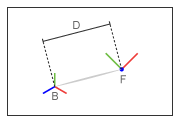# Distance Constraint

Fixed distance between two frame origins

## Library

Constraints

•## Description

This block applies a fixed distance between the origins of the base and follower port frames. The frames lose one translational degree of freedom with respect to each other. The constraint distance between the frame origins, labeled D in the figure, must be greater than zero.The block provides constraint force sensing in the form of a vector or a signed magnitude. These quantities are contained in physical signals that the block outputs through Simscape™ PS ports. The constraint force is the force required to maintain the specified distance between the port frame origins.

## Parameters

Distance

Constraint distance between the base and follower frame origins. The distance must be greater than zero. For a distance of zero, use a Spherical Joint or Gimbal Joint block instead. The default value is `1` m.

### Constraint Force Sensing

Select whether to compute and output the distance constraint force vector and its signed magnitude. The distance constraint force is the force that the block must apply in order to maintain the distance you specify between the base and follower port frames.

Direction

Constraint forces act in pairs. As expressed by Newton’s third law of motion, if the base port frame exerts a constraint force on the follower port frame, then the follower port frame must exert an equal and opposite force on the base port frame. Select which of the two constraint forces to sense:

• `Follower on Base` — Sense the constraint force that the follower port frame exerts on the base port frame.

• `Base on Follower` — Sense the constraint force that the base port frame exerts on the follower port frame.

Resolution Frame

The block expresses the constraint force vector in terms of its Cartesian vector components. The splitting of a vector into vector components is known as vector resolution. The frame whose axes define the vector component directions is known as the resolution frame. Select whether to resolve the constraint force vector in the base or follower port frame.

Force Vector

Compute and output the Cartesian components of the distance constraint force vector. The output signal is a three-dimensional vector, [fx, fy, fz].

Signed Force Magnitude

Compute and output the magnitude of the distance constraint force, including its sign.

## Ports

The block provides two frame ports:

• B — Base frame port

• F — Follower frame port

In addition, the block provides two physical signal output ports:

• f — Distance constraint force vector

• fm — Signed magnitude of the distance constraint force

## Version History

Introduced in R2012a